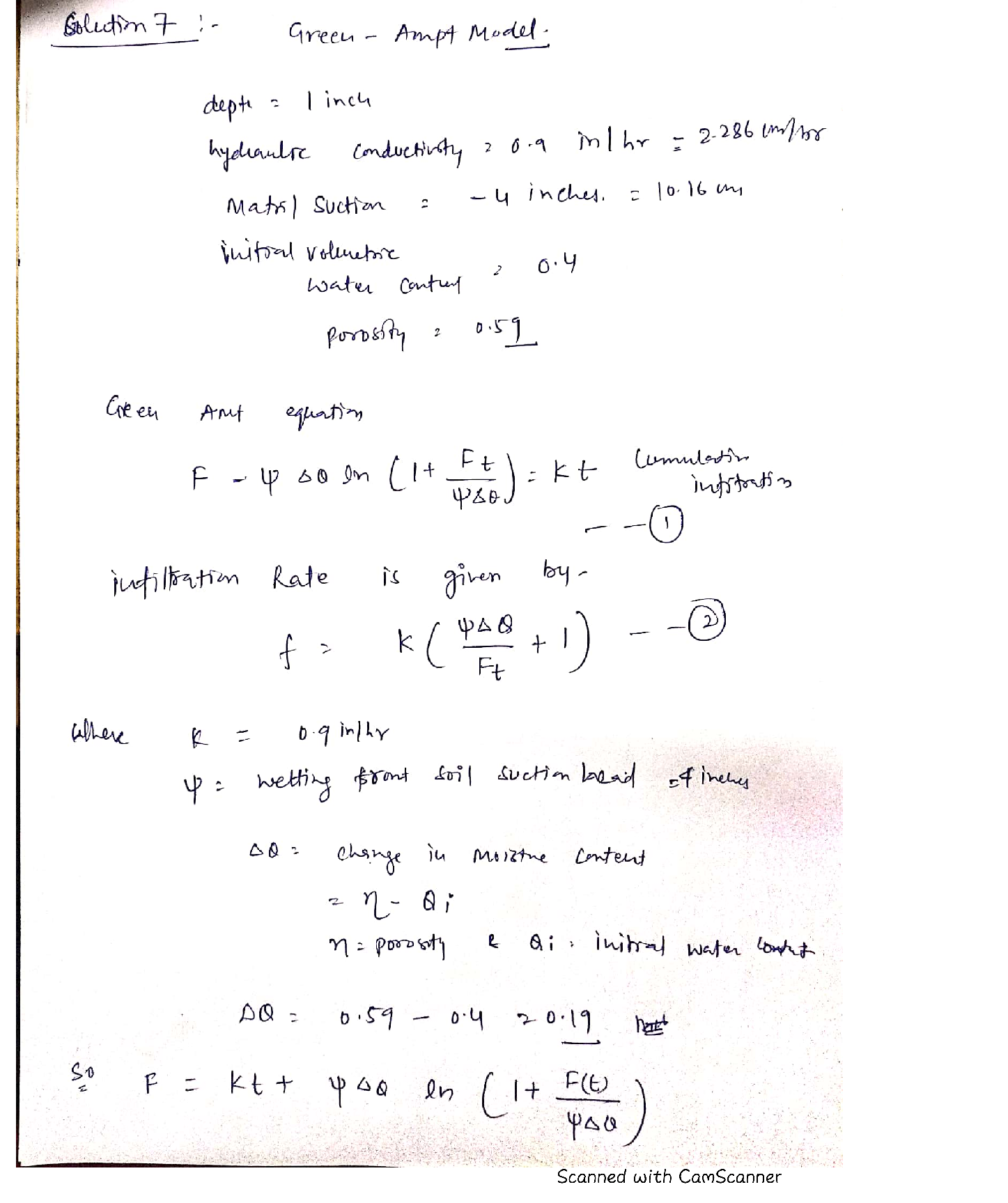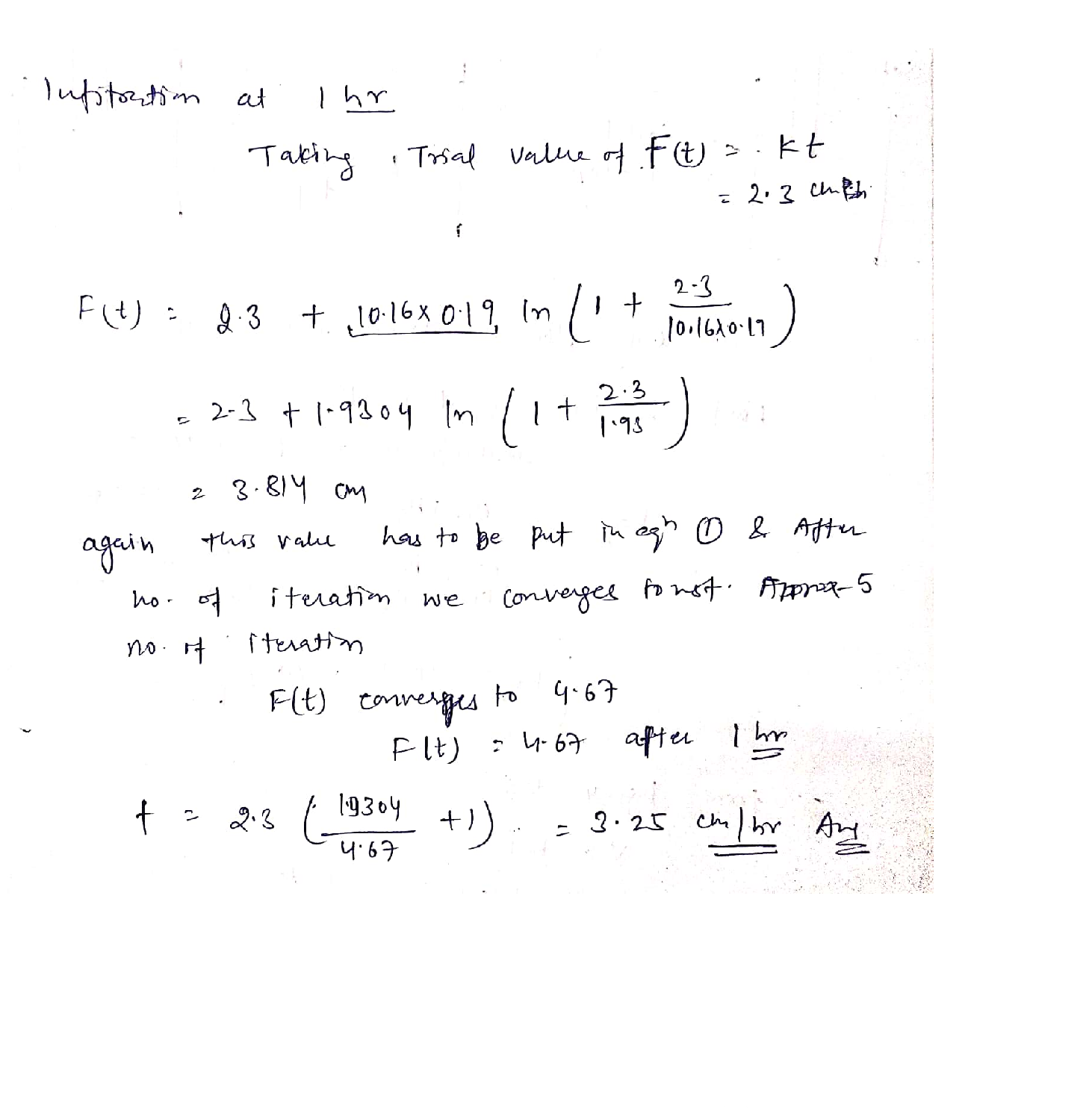Question

Hydrology

. Using the Green-ampt model, what is the infiltration rate if the saturation zone has reached 1 inch in depth, the hydraulic conductivity is 0.9 inches/hour, the matril suction is -4 inches, the initial volumetric water content is 0.4, and the porosity is 0.59? (5 pts)Verified### Question 40869Hydrology

IL An unconfined aquifer flows horizontally 2000 R in a soil with a K = 10 ft/day, followed hy 3000 ft in a soil with a K of 9 fi/day. The water level at the beginning of this reach is50 fl and at the end of this reach (5000 ft away) is 45 ft, measured above the bottom of the confining layer. The flow is horizontal and one-dimensional. What is the flow rate per unit width? (12 pt)

### Question 40868Hydrology

. Using the Green-ampt model, what is the infiltration rate if the saturation zone has reached 1 inch in depth, the hydraulic conductivity is 0.9 inches/hour, the matril suction is -4 inches, the initial volumetric water content is 0.4, and the porosity is 0.59? (5 pts)

### Question 40867Hydrology

. Using the 1-hour UH hydrograph data below, calculate the peak flowrate for a storim event which has 0.25 inches of runoff in the first hour, and 0.5 inches in the second hour and 0.4 inches in a third hour (you do not need to show the full hydrograph). 10 pts

### Question 40866Hydrology

For a given soil, you find the particle density is 2.3 kg/L, the dry bulk density is 1.55kg/L, and the volumetric water content is 0.4.
a. Porosity:
b. Saturation:
g. Fraction of volume that is particles
d. Fraciton of mass that is particlesc. Fraction ofu
c.Fraction of mass that is water
c. Fraction of volume that is water
f. Fraction of volume that is air

### Question 40864Hydrology

You measure in the soil above that the initial infiltration rate is 2.7 inches/hur, and the final capacity is 0.6 inches per hour, and it has a time constant of 0.25 hr-1. Assuming sufficient rainfall for maximum infilitration rates, what is the infiltration rate at 1 hour using Horton's model (5 pts)?

### Question 40862Hydrology

If well watered grass has a evapotranspiration of 0.6 inches/day, but you have com with a crop coefficient of 1.6 and 40% available water content, what is the amount of evapotranspiration you expect for your corn? (3 pts)
a. 0.38 inches b. 0.96 inches c. 0.58 inches d. 1.6 inches

### Question 40665Hydrology

Calculate the hydrodynamic reaction of the curved wall of an open horizontal rectangular channel,knowing the volumetric flow rate Qy [m³/s], width b [m] and the water filling height h [m]. The width of the outlet channel is 0,7b [m]. For the calculations, take:
b=0,3+0,01 \cdot N r[m]=
Q_{V}=0,12+0,01 \cdot N r\left[m^{3} / s\right]=
h=0,2+0,01 \cdot N r[m]=
\rho=1000\left[\mathrm{~kg} / \mathrm{m}^{3}\right]

### Question 40315Hydrology

\text { Route the given hydrograph and determine the stage (feet) and outflow (cfs) at various times. }

### Question 40314Hydrology

5. What is the value (cfs) at elevation 5.0 feet (top of pond) of the stage-storage-discharge curve (deltat=0.1 hours) assuming a detention pond with volume, weir, orifice, and infiltration as identified in Questions 1 thru 4? (a) < 750 cfs (b) 750-1500 cfs (c)1500-2500 cfs (d) > 2500 cfs
\text { Stage-storage-discharge curve (cfs) }-25 / d t+0
Storage (S) is the total volume (cf) at the elevation in question
Delta time (dt) is the time step (seconds) which is given
Outflow (O) is the total outflow rate (cfs) at the elevation in quêstion

### Question 40313Hydrology

\text { 4. What is the flowrate (cfs) due to infiltration at elevation } 5.0 \text { feet if } K \text { (ft/day) }=5.0 ? \text { (a) }<0.5 \text { cfs (b) } 0.5-2 \text { cfs (c) } 2-4 \text { cfs (d) }>4 \text { cfs }
\text { Darcy's Law- Q (cfs) }=K^{\star} i^{\star} A
A (sf) is the area at the elevation in question
• i = dH/dL = 1%3D
K (ft/s) is the hydraulic conductivity

### Submit query